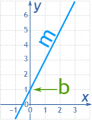Joined Apr 2, 2021
22
Hello,
I found a 3-axial accelerometer with +/-500g that has a +/-1.25V range. The bias voltage (0g) is at half of the reference given (It does not give negative voltage).
If I'm supplying it with 3.3V, the bias voltage will be at 1.65V, so the range will be nominally 0.4V to 2.9V.
Can anyone help me with matching the readings of the ADC to obtain -500g at 0.4V and +500g at 2.9?

#### ericgibbs

Joined Jan 29, 2010
16,808
As the ADC is SPI, which MCU are you using.?
E

Joined Apr 2, 2021
22
Hello Eric
I am using RaspberryPi that has an SPI connection

Joined Apr 2, 2021
22
Yes, this is what I am willing to do, but I don't know how to do it.
If I use this formula, I will obtain 496 at 0.4V, and 3600 at 2.9V.
How do I obtain -500 for 0.4V and 500 for 2.9V ?

#### ericgibbs

Joined Jan 29, 2010
16,808
hi j,
Very basic program. the first entry in the bracket is the Value.

C-like:
float Acc1= 0;

void setup() {
Serial.begin(9600);
}

void loop() {

Acc1 = map(0.4,0.4, 2.9, -500, 500);

// Serial.print("Acc1= ");
Serial.println(Acc1);

delay(1000);

Acc1 = map(2.9,0.4, 2.9, -500, 500);

// Serial.print("Acc1= ");
Serial.println(Acc1);

delay(1000);

}

#### Attachments

Joined Apr 2, 2021
22
Can you please explain what did the map function did? And why is there 2 of them?
Sorry for my basic knowledge

#### ericgibbs

Joined Jan 29, 2010
16,808
hi j,
The MAP function will not accept decimal value, so for demonstration I have multiplied by 10

I used different values in the last Sketch to show the limits

This version increments the Value and outputs the result of the MAP operation.

The simple sketch just demonstrates the method, you will have to fine tune it.

What are the Lowest and Highest values coming from the ADC via the SPI.??

E

C-like:
float Acc1= 0;
float Val1= 0;
void setup() {
Serial.begin(9600);

Val1= 4; // Note Decimal Value *10 into Integer
}

void loop() {

Acc1 = map(Val1,4, 29, -500, 500);

Serial.print(Val1);
Serial.print(" ");
Serial.println(Acc1);

Val1=Val1+1;
delay(500);
}

#### Attachments

Joined Apr 2, 2021
22
Hello
So it is simple math, thanks for the clarification.

What are the Lowest and Highest values coming from the ADC via the SPI.??
In fact, I did not receive the components, so I am just preparing for later. And plus, I have a report to make about the machine.

I have another question please. What about filtering my output? Does it really matter if I'm oversampling? And if it matters, do I need to build one by myself or there are pre built anti alias (low pass filter)

#### ericgibbs

Joined Jan 29, 2010
16,808

#### Attachments

• 118 KB Views: 2
Last edited:

#### MrChips

Joined Oct 2, 2009
27,702
You can use the equation of a straight line.

y = mx + b

where
y is the dependent variable, your acceleration
m is the slope
b is the intercept on the y-axis at x = 0You have two data points:
(x1, y1) = (0.4, -500)
(x2, y2) = (2.9, 500)

If you desire, you may replace your x values with ADC values

Calculate the slope m.
m = (y2 - y1) / (x2 - x1) = (500 - (-500)) / (2.9 - 0.4) = 1000 / 2.5 = 400

Calculate the intercept b using either of the two given data points and your calculated value of m.
b = y - mx

for example, using (x2, y2)
b = y2 - mx2 = 500 - 400*2.9 = -660

double check using (x1, y1)
b = y1 - mx1 = -500 - 400*0.4 = -660

Hence the equation is
y = 400x - 660

•Joined Apr 2, 2021
22
You can use the equation of a straight line.

y = mx + b

where
y is the dependent variable, your acceleration
m is the slope
b is the intercept on the y-axis at x = 0

View attachment 246437

You have two data points:
(x1, y1) = (0.4, -500)
(x2, y2) = (2.9, 500)

If you desire, you may replace your x values with ADC values

Calculate the slope m.
m = (y2 - y1) / (x2 - x1) = (500 - (-500)) / (2.9 - 0.4) = 1000 / 2.5 = 400

Calculate the intercept b using either of the two given data points and your calculated value of m.
b = y - mx

for example, using (x2, y2)
b = y2 - mx2 = 500 - 400*2.9 = -660

double check using (x1, y1)
b = y1 - mx1 = -500 - 400*0.4 = -660

Hence the equation is
y = 400x - 660
Thank you! It's very clear now

If you decide to filter, post the signal details and I will use LTSpice to check the filter.

E
My sensor has 2-6000Hz frequency response.
In the norm (EN1177) they said we need and 12-bit ADC, sampling at over than 20KSPS, and an anti-alias filter of -30dB at the half of the sampling frequency.
Is there a problem if I sample at 1MSPS?
When building an anti-alias filter, should I consider half of the sampling frequency like stated by the norm, so at 500KSPS?
Or can I build it at 10000Hz to eliminate other frequencies.
The ADC selection is not definitive, so please consider any ADC with the sampling rate of >20000Hz.

#### ericgibbs

Joined Jan 29, 2010
16,808
hi j,
What is the Accelerometer attached too and how are you using the output data.?
Look at the over sampling Program options in the ADC. d/s 8.3.5

E

Joined Apr 2, 2021
22
The accelerometer is placed in a fake head for drop tests.
The output data is going to LabVIEW using linx and we use the data to calculate the velocity and height

#### ericgibbs

Joined Jan 29, 2010
16,808